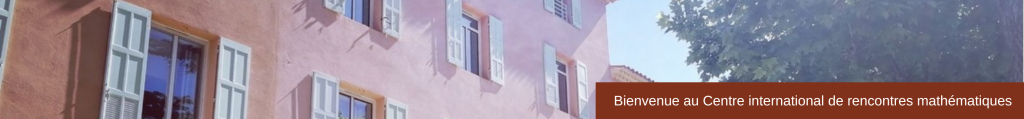RESEARCH IN PAIRS
﻿Normal Hilbert Coefficients and Itoh’s Conjecture
3-7 July 2017
 The focus of our proposed project is to understand the significance of the asymptotic properties encoded in the Hilbert function of the integral closure filtration fIng of a zero dimensional ideal in a local Cohen-Macaulay ring. There has been quite some interest in Commutative Algebra in relating bounds for the normalized Hilbert coefficients and the depth of the associated graded ring of the normal filtration of a zero dimensional ideal. The tool usually used is the local cohomology of the normalization of the Rees algebra, in particular one needs to understand the vanishing properties of this object. Our main goal is to prove Itoh’s conjecture, which is open since 1992. This conjecture states that the vanishing of the third normalized Hilbert coefficient is equivalent to the equality In+2 = JnI2, where J is any ideal generated by a regular sequence such that J = I. The last statement is very similar in spirit to Huneke’s and Itoh’s celebrated result on integral closures of powers of complete intersections. It also implies the Cohen-Macaulayness of the Rees ring and the associated graded ring of the integral closure filtration with vanishing third Hilbert coefficient. Participants Chennai Mathematical Institute)University of Notre Dame)University of Genoa) Sponsor